# Determine Solutions to Equations and Inequalities Through Substitution Lesson Plan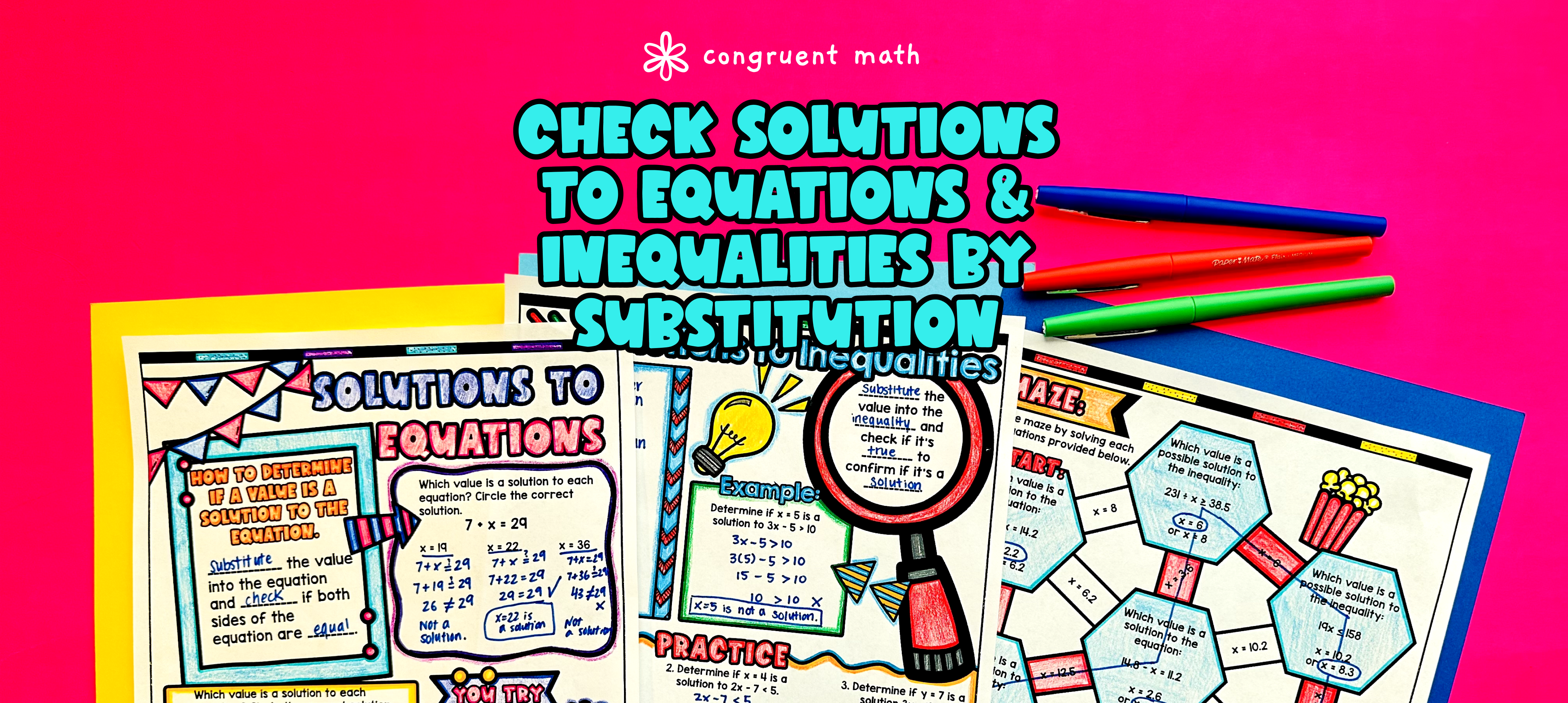Pin This

## Overview

Ever wondered how to teach how to use substitution to determine whether a given number in a specified set makes an equation or inequality true in an engaging way to your 6th-grade students? Looking for way to cover common core standards CCSS 6.EE.B.5?

In this lesson plan, students will learn about substitution in equations and inequalities and their real-life applications. Through artistic, interactive guided notes, checks for understanding, a doodle & color by number activity, and a maze worksheet, students will gain a comprehensive understanding of using substitution to determine solutions for equations and inequalities.

The guided notes provide a structured approach to teaching the topic, integrating checks for understanding to ensure that students are on the right track. Following the guided notes, students will have the opportunity to practice their skills with a fun color by code activity, a maze worksheet, and additional problem sets.

The lesson culminates with a real-life application, where students will read and write about the real-life uses of using substitution to solve mathematical problems. This application will help students see the relevance of the topic in their everyday lives.

## Get the Lesson Materials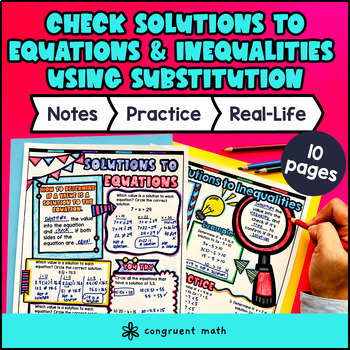\$4.25

## Learning Objectives

After this lesson, students will be able to:

• Use substitution to determine whether a given number in a specified set makes an equation true

• Use substitution to determine whether a given number in a specified set makes an inequality true

## Prerequisites

Before this lesson, students should be familiar with:

• Basic operations with positive whole numbers and decimals

• Understanding the concept of variables and their role in equations

• Basic knowledge of the order of operations

• Substitution

• Equations

• Inequalities

• Solutions

• Variables

• Coefficients

• Constants

• Expressions

## Procedure

### Introduction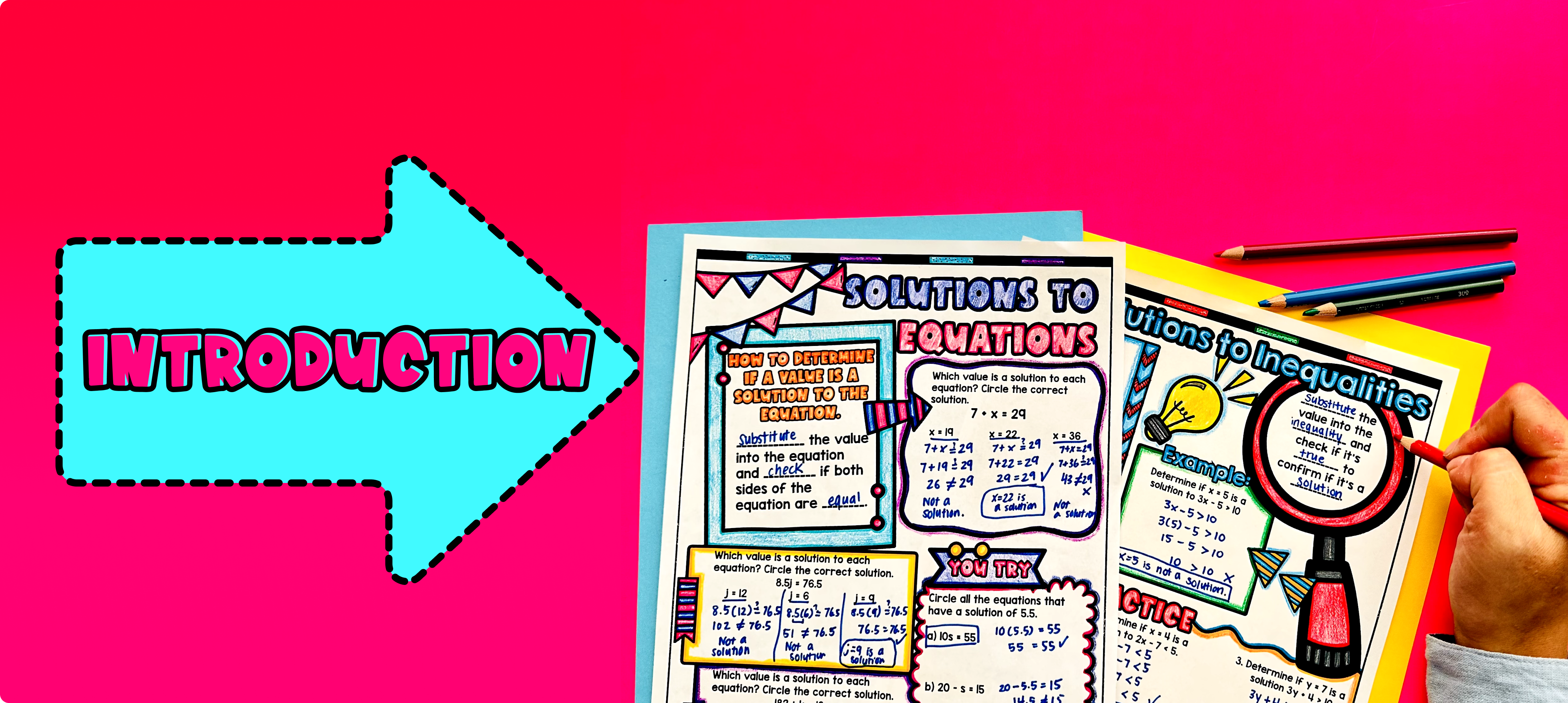Pin This

As a hook, ask students why solving equations and inequalities is important in real life. Refer to the last page of the guided notes for ideas.

Use the first page of the guided notes to introduce the concept of substitution and how it can be used to determine solutions for equations. Then use the second page of the guided notes to introduce how to determine solutions for inequalities by substitution. Walk through the key points of using substitution, including replacing a variable with a given number and checking if the equation or inequality is true.

Based on student responses, reteach concepts that students need extra help with. If your class has a wide range of proficiency levels, you can pull out students for reteaching, and have more advanced students begin work on the practice exercises.

### Practice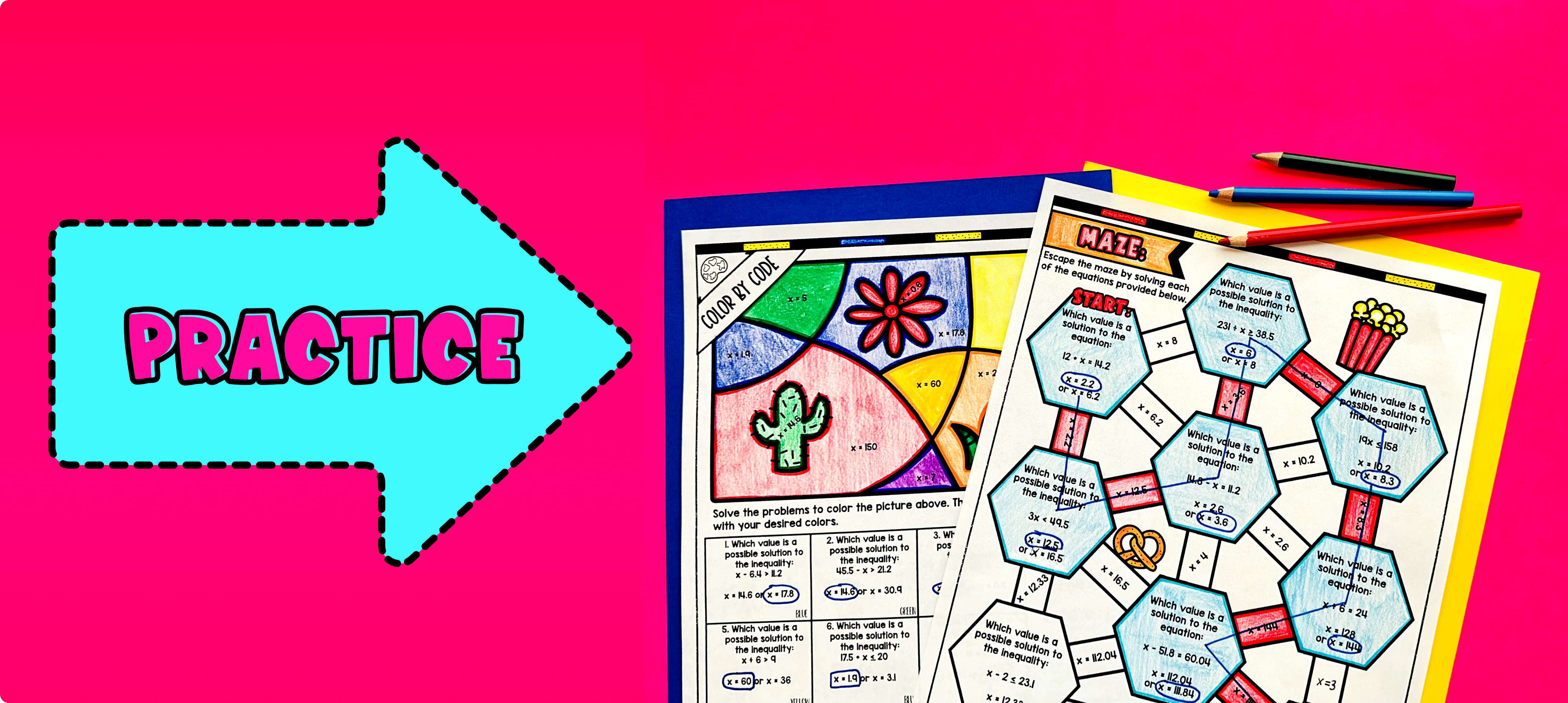Pin This

Have students practice using substitution to determine solutions for equations and inequalities using the practice section included on page 1-2 of the guided notes. Walk around the classroom to answer any student questions and provide support as needed.

Fast finishers can further solidify their understanding by completing the maze activity (page 3) and color by number activity (page 4) included in the guided notes resource. You can assign it as homework for the remainder of the class.

### Real-Life Application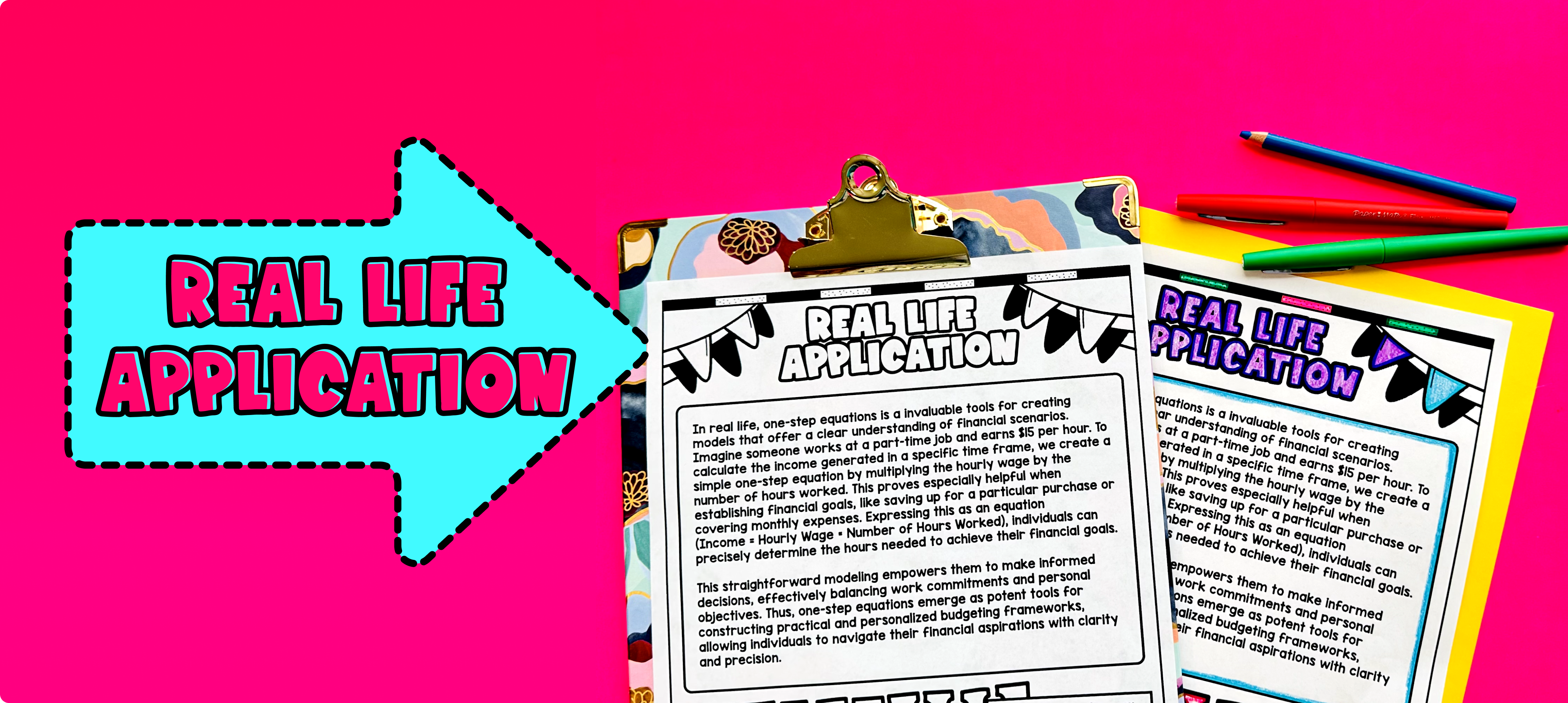Pin This

Use the last page of the guided notes to bring the class back together, and introduce the concept of real-world application of using substitution to determine solutions for equations and inequalities. Provide examples of situations in which this concept can be applied in daily life, such as budgeting or finding the best deals while shopping. Discuss how substitution can be used to solve equations or inequalities to find the value of a variable that satisfies a given condition.